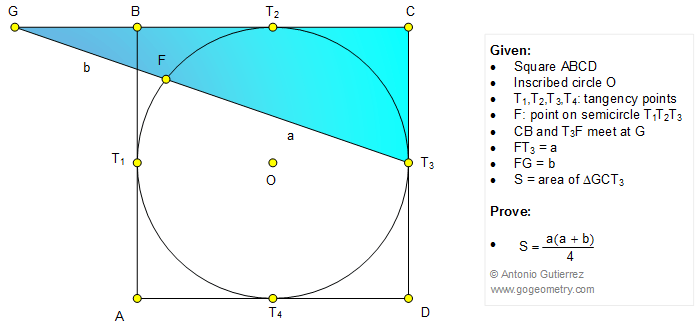Online Geometry Problem 1078: Square, Inscribed Circle, Tangent, Triangle, Area. Level: High School, Honors Geometry, College, Mathematics Education

< PREVIOUS PROBLEM  |  NEXT PROBLEM >

 In the figure ABCD is a square and circle O is tangent to AB, BC, CD, and AD at T1, T2, T3, and T4, respectively. F is a point on the semicircle T1T2T3, and lines CB and FT3 meet at G. If FT3 = a, FG = b, and S is the area of triangle GCT3, prove that S = a(a+b)/4.Home | Search | Geometry | Problems | All Problems | Open Problems | Visual Index | 10 Problems | 1071-1080 | Triangle | Square | Circle | Areas | Email | by Antonio Gutierrez Solution / Comment to Geometry Problem 1078 Last updated: Jan 31, 2015